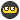## Recommended Posts

Ok I have a relatively simple problem yet the solution escapes meI'll spare ya the details of my script, just get down to the problem. I need to be able to determine which number is smallest in a list, and select the point that number refers to. The list is in a format like this,

point#1,distance,point#2,distance, etc.

The list's lenght changes, but it's composed of pair values (point#,distance)

so an example would be,

1,80,2,100,3,60,4,150

The smallest distance is 60 from the above example, so therefore the script should return the point number 3. I have no idea how to make the script determine which of the distance numbers given in that string is the smallest and return the point number it refers to. Unfortunately in my case, this string format is the only option.

Anyone can help me out with this?

Edited by valkk

##### Share on other sites

Because your list is structured, the simplest way is using StringSplit and then building new array with every second value from splitteed array. Something like:

```Dim \$list = "1,80,2,100,3,60,4,150", \$cnt

\$aList = StringSplit(\$list, ",")

For \$cnt = 0 to UBound(\$aList)/2-1
Next

Dim \$min = \$aDistList, \$num = 1

For \$cnt = 1 to UBound(\$aDistList)-1
\$num = \$cnt + 1
Endif
Next

MsgBox (0, "title", "Min distance (" & \$min & ") is point " & \$num)```

But it will work if you points are ordered (1,2,3... etc). If they numbers are mixed you need a bit more complex:

```\$list = "1,80,2,100,3,60,4,150"

\$aList = StringSplit(\$list, ",")

For \$cnt = 0 to UBound(\$aList)/2-1
Next

For \$cnt = 1 to UBound(\$aDistList)-1
Endif
Next

MsgBox (0, "title", "Min distance (" & \$min & ") is point " & \$num)```
Edited by Kot

##### Share on other sites

```\$anValues = StringSplit (\$sString, ",")
;Prep Min markers
\$nMinDistance = 99999
\$nMinPoint = 0
for \$nX = 2 to \$anValues step 2
if \$anValue[\$nX] < \$nMinDistance then
;Found a new Min
\$nMinDistance = \$anValue[\$nx]
\$nMinPoint = \$anValue[\$nx-1]
end if
next
;return results
\$sMsg = "Min Distance = " & \$nMinDistance & @LF & "Min Point = " & \$nMinPoint
Msgbox (0,"Min", \$sMsg )```

##### Share on other sites

Thank you for supportI thougt I'll try mix your two examples ^^ and it works but during one test I got some strange result. At one point I have this statement, If 80 < 130 Then, which is true 80 is smaller then 130 BUT it skips it. Here's my example,

```\$listchoices = "17,130,22,80";

\$listnum = stringsplit(\$listchoices,",");

\$Smallest = 10000;
For \$count = 1 to (\$listnum/2);
If \$listnum[\$count*2] < \$Smallest Then
\$Smallest = \$listnum[\$count*2]
\$point = \$listnum[(\$count*2)-1];
EndIf
Next
msgbox(0, "test", "Choosen:"&\$point);```

For that particular list of values, it chooses #17 which refers to 130. This is wrong, 80 is smaller then 130. After playing around I learned that IF statement was getting skipped, leading me to conclude that it thinks 80 is NOT smaller then 130. I put a msgbox returning the exact values of the IF statement, \$listnum[\$count*2] < \$Smallest, and it returned If 80 < 130. Umm doesn't take a genious to realize that's true, yet the script thinks it's false.Here's the funny thing. If I replace \$listnum[\$count*2] with the number 80, as it should be, it works; the statement is true. What's weird is \$listnum[\$count*2] is equal to 80 on the 2nd loop.

Edited by valkk

##### Share on other sites

your script does a string compare in stead of a number compare..

this will do it:

```\$listchoices = "17,130,22,80";

\$listnum = stringsplit(\$listchoices,",");

\$Smallest = 10000;
For \$count = 1 to (\$listnum/2);
If number(\$listnum[\$count*2]) < number(\$Smallest) Then
\$Smallest = \$listnum[\$count*2]
\$point = \$listnum[(\$count*2)-1];
EndIf
Next
msgbox(0, "test", "Choosen:"&\$point);```

Live for the present,
Dream of the future,
Learn from the past.##### Share on other sites

your script does a string compare in stead of a number compare..

this will do it:

Ohhhhhhhhh. That I didn't know, guess I thought it would consider it as a number automatically.

##### Share on other sites

Ohhhhhhhhh.  That I didn't know, guess I thought it would consider it as a number automatically.

<{POST_SNAPBACK}>

I wish

Returns the numeric representation of an expression.

Number ( expression )

Became my favorite section of the help file while writing a complicated generatesalesreport script

Rick

Only \$2.00 with Resale Rights How to Block Better for Martial Artists and NonMartial Artistshttp://kirkhamsebooks.com/MartialArts/Bloc...tterEbook_m.htm

## Create an account

Register a new account

• ### Recently Browsing   0 members

×

• Wiki

• Back

• #### Beta

• Git
• FAQ
• Our Picks
×
• Create New...